#### 期刊菜单

Study on the Numerical Simulation of Hydro-Mechanical Coupling of Complex Fracture Network Propagation in Shale Gas Reservoirs
DOI: 10.12677/APF.2022.121001, PDF, HTML, XML, 下载: 35  浏览: 91

Abstract: The commercial development of shale gas reservoirs mainly depends on hydraulic fracturing technology. Accurate prediction of fracture network propagation in shale gas reservoirs is of great significance for the optimization design of fracturing schemes and the calculation of post-fracturing productivity. In this paper, considering the hydro-mechanical coupling effect, the mathematical model of complex fracture network propagation in shale gas reservoirs is established, and the finite element method and finite volume method are used to discretize the stress field and pressure field. The accuracy of the model and algorithm is verified by comparing them with the analytical solution of the KGD model, and then the influence of horizontal stress difference and perforation cluster number on the fracture pattern is analyzed, and the fracture network propagation law in shale gas reservoirs is expounded, which has significance for guiding the optimal design of fracturing in shale gas reservoirs.

1. 引言

2. 裂缝扩展数学模型

2.1. 岩体变形

$\nabla \cdot \sigma +f=0$ (1)

${\sigma }^{\prime }=\sigma +\alpha {p}_{\text{m}}I$ (2)

${\sigma }^{\prime }=D\epsilon$ (3)

2.2. 流体流动

$\frac{1}{M}\frac{\partial {p}_{\text{m}}}{\partial t}+\alpha \frac{\partial {\epsilon }_{\text{v}}}{\partial t}-\nabla \cdot \left(\frac{{k}_{\text{m}}}{\mu }\nabla {p}_{\text{m}}\right)={\delta }_{\text{mt}}\frac{{q}_{\text{t}}}{{V}_{m}}+{\delta }_{\text{mb}}\frac{{q}_{\text{b}}}{{V}_{m}}$ (4)

$\frac{\partial w}{\partial t}-\nabla \cdot \left(\frac{{w}^{3}}{12\mu }\nabla {p}_{\text{f}}\right)+\frac{{q}_{\text{t}}}{{S}_{\text{f}}}+\frac{{q}_{\text{b}}}{{S}_{\text{f}}}={Q}_{\text{f}}$ (5)

${q}_{\text{t}}=c\left({p}_{\text{f}}-{p}_{\text{t}}\right)$ (6)

${q}_{\text{b}}=c\left({p}_{\text{f}}-{p}_{\text{b}}\right)$ (7)

2.3. 裂缝扩展准则

$\mathrm{max}\left\{\frac{〈{t}_{\text{n}}〉}{{t}_{\text{n}}^{0}},\frac{{t}_{\text{s}}}{{t}_{\text{s}}^{0}},\frac{{t}_{\text{t}}}{{t}_{\text{t}}^{0}}\right\}\ge \text{1}$ (8)

$D=\frac{{\delta }^{\text{f}}\left({\delta }^{\mathrm{max}}-{\delta }^{0}\right)}{{\delta }^{\mathrm{max}}\left({\delta }^{\text{f}}-{\delta }^{0}\right)}$ (9)

3. 数值求解方法

$\underset{\Omega }{\int }\nabla u:{\sigma }^{\prime }\text{\hspace{0.17em}}\text{d}\Omega -\underset{\Omega }{\int }\nabla u\cdot \alpha {p}_{\text{m}}I\text{\hspace{0.17em}}\text{d}\Omega +\underset{{\Gamma }_{\text{f}}}{\int }〚u〛\cdot \left({t}_{\text{c}}-{p}_{\text{f}}{n}_{\text{f}}\right)\text{d}\Gamma =\underset{{\Gamma }_{\text{t}}}{\int }u\cdot \stackrel{¯}{t}\text{d}\Gamma +\underset{\Omega }{\int }u\cdot f\text{\hspace{0.17em}}\text{d}\Omega$ (10)

$\begin{array}{l}-\underset{\Delta t}{\int }\underset{{\Omega }^{e}}{\int }\nabla \cdot \left(\frac{{k}_{\text{m}}}{\mu }\nabla {p}_{\text{m}}\right)\text{\hspace{0.17em}}\text{d}\Omega \text{\hspace{0.17em}}\text{d}t+\underset{\Delta t}{\int }\underset{{\Omega }^{e}}{\int }\frac{1}{M}\frac{\partial {p}_{\text{m}}}{\partial t}\text{\hspace{0.17em}}\text{d}\Omega \text{\hspace{0.17em}}\text{d}t+\underset{\Delta t}{\int }\underset{{\Omega }^{e}}{\int }\alpha \frac{\partial {\epsilon }_{\text{v}}}{\partial t}\text{\hspace{0.17em}}\text{d}\Omega \text{\hspace{0.17em}}\text{d}t\\ =\underset{\Delta t}{\int }\underset{{\Omega }^{e}}{\int }\left({\delta }_{\text{mt}}\frac{{q}_{\text{t}}}{{V}_{\text{m}}}+{\delta }_{\text{mb}}\frac{{q}_{\text{b}}}{{V}_{\text{m}}}\right)\text{\hspace{0.17em}}\text{d}\Omega \text{\hspace{0.17em}}\text{d}t\end{array}$ (11)

4. 计算结果分析

4.1. 模型验证

$w=\frac{4\left(1-v\right)L}{\pi G}\left[{\int }_{{f}_{L}}^{1}\frac{{f}_{2}\text{d}{f}_{2}}{\sqrt{{f}_{2}^{2}-{f}_{L}^{2}}}{\int }_{0}^{{f}_{2}}\frac{p\left({f}_{1}\right)\text{d}{f}_{1}}{\sqrt{{f}_{2}^{2}-{f}_{1}^{2}}}-\frac{\pi }{2}{\sigma }_{\mathrm{min}}\sqrt{1-{f}_{L}^{2}}\right]$ (12)

${p}_{\text{w}}-p=\frac{12\mu {q}_{0}L}{h}{\int }_{{f}_{Lw}}^{{f}_{L}}\frac{\text{d}{f}_{L}}{{w}^{3}}$ (13)

Geertsma和De Klerk推导得到模型的近似解析解，其中裂缝半长L、井筒处缝宽w0随时间t的演化关系为：

$L\left(t\right)=\text{1}\text{.078}{\left(\frac{{E}^{\prime }{q}_{0}^{3}}{\mu }\right)}^{1/6}{t}^{2/3}$ (14)

${w}_{0}\left(t\right)=2.36{\left(\frac{\mu {q}_{0}^{3}}{{E}^{\prime }}\right)}^{1/6}{t}^{1/3}$ (15)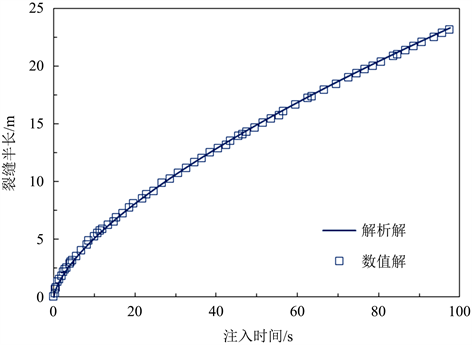Figure 1. Comparison of fracture half-length between numerical and analytical solutions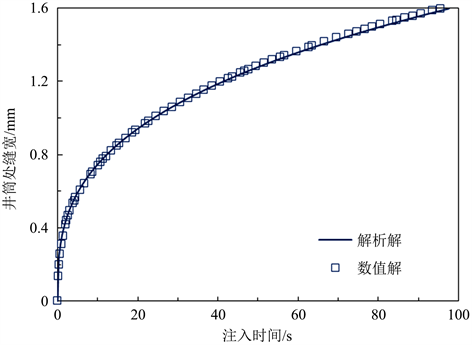Figure 2. Comparison of fracture width at the inlet of wellbore between numerical and analytical solutions

4.2. 水平应力差的影响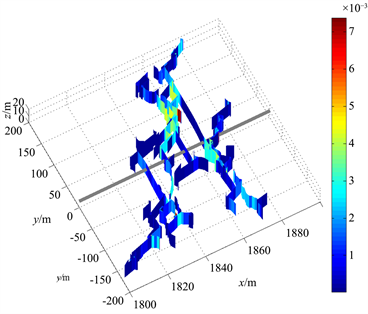(a)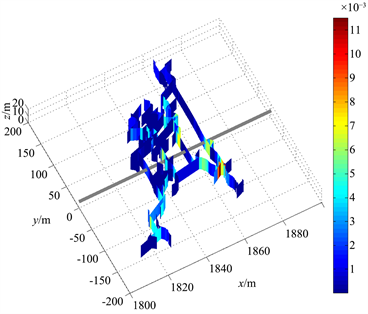(b)(c)

Figure 3. Results of hydraulic fracture propagation under different conditions of stress difference: (a) Δσ = 5 MPa; (b) Δσ = 7 MPa; (c) Δσ = 9 MPa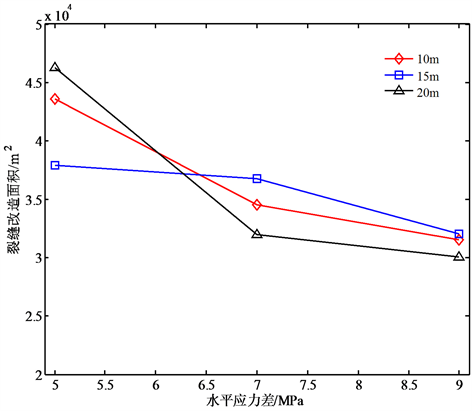Figure 4. Results of fracture stimulated area under different conditions of stress difference

4.3. 射孔簇数的影响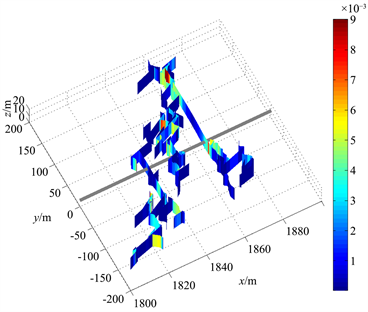(a)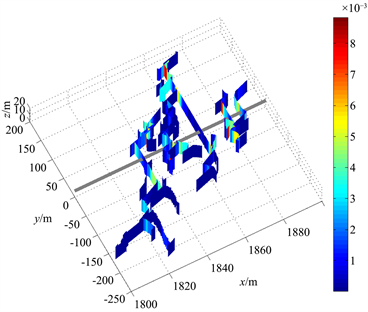(b)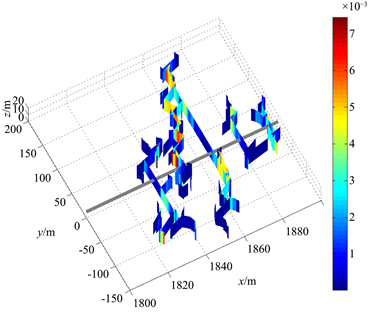(c)

Figure 5. Results of hydraulic fracture propagation under different conditions of cluster number: (a) Three clusters; (b) Four clusters; (c) Five clusters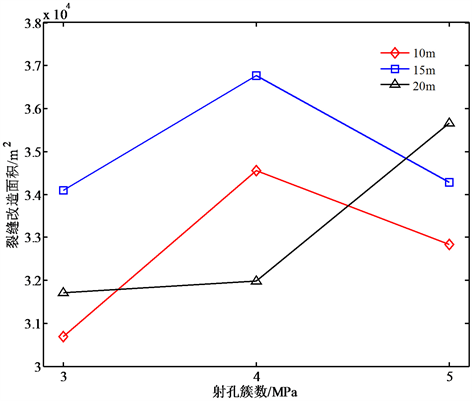Figure 6. Results of fracture stimulated area under different conditions of cluster number

5. 结论

1) 多簇压裂裂缝扩展存在应力阴影作用，水平应力差越小，则应力阴影作用越强，裂缝扩展形态越集中，裂缝改造面积越小；当水平应力差大于7 MPa时，选择簇间距为15 m可获得最大的裂缝改造面积；

2) 多簇射孔压裂，射孔簇数越多，应力阴影作用越强，沟通的天然裂缝越多，形成的裂缝形态越分散；当簇间距小于20 m时，随着射孔簇数增大，裂缝改造面积先增大后减小。

NOTES

*通讯作者。

  姚军, 孙海, 黄朝琴, 等. 页岩气藏开发中的关键力学问题[J]. 中国科学(物理学, 力学, 天文学), 2013, 43(12): 1527-1547.  庄茁, 柳占立, 王涛, 等. 页岩水力压裂的关键力学问题[J]. 科学通报, 2016, 61(1): 72-81.  Chen, Z.R. (2012) Finite Element Modelling of Viscosity-Dominated Hydraulic Fractures. Journal of Pe-troleum Science and Engineering, 88, 136-144. https://doi.org/10.1016/j.petrol.2011.12.021  曾青冬, 姚军. 基于扩展有限元的页岩水力压裂数值模拟[J]. 应用数学和力学, 2014, 35(11): 1239-1248.  Wu, K. and Olson, J. (2015) Simultaneous Multifracture Treatments: Fully Coupled Fluid Flow and Fracture Mechanics for Horizontal Wells. SPE Journal, 20, 337-346. https://doi.org/10.2118/167626-PA  顾颖凡, 卢毅, 刘兵, 等. 基于离散元法的水力压裂数值模拟[J]. 高校地质学报, 2016, 22(1): 194-199.  刘国威, 李庆斌, 梁国贺. 动力水力压裂的相场模拟方法[J]. 岩石力学与工程学报, 2017, 36(6):1400-1412.  张钰彬, 黄丹. 页岩水力压裂过程的态型近场动力学模拟研究[J]. 岩土力学, 2019, 40(7): 2873-2881.  Geertsma, J. and De Klerk, F. (1969) A Rapid Method of Predicting Width and Extent of Hydraulically Induced Fractures. Journal of Petroleum Technology, 21, 1571-1581. https://doi.org/10.2118/2458-PA